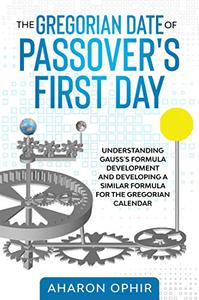# Welcome!

By registering with us, you'll be able to discuss, share and private message with other members of our community.

# eBooksThe Gregorian Date of Passover's First Day

##### Well-known memberEnglish | 2021 | ISBN: N/A | ASIN: B08VRJ9TV5 | 182 pages | EPUB/PDF | 6 Mb​

The book deals with determining the Christian date of the first day of Passover.The reader is first presented, by the book "The Gregorian date of Passover's first day", with a mathematical analysis of the well-known Gaussian formula. This presentation includes a simple and step-by-step explanation of how this formula is obtained. The book explains the logic behind the use of each parameter. The book also explains how this formula can be calculated so that the result is accurate.In the part that follows, the book constructs a similar formula that calculates the date in the Gregorian calendar of the first day of Passover. Here, too, the book explains in details, how the formula is obtained, in simplicity, and in a step-by-step method.In the last part, the book addresses the inaccuracies that also exist in the Gregorian calendar. The book formulates the desired amendment, in the author's opinion, for an improved Gregorian calendar. In addition, the book also presents a formula for calculating the date, of the first day of Passover, in this calendar.Understanding how to arrive at this formula will also make it possible to teach it in schools in a simple and organized way.I really hope you will like this ebook. Enjoy!Code:
``https://rapidgator.net/file/9370237406c4ff15d30319721405c855``

Code:
``https://nitro.download/view/648ACC67A5CA1F6``

Code:
``https://1dl.net/afzv2iscoarw/BaDshaH.B08VRJ9TV5.rar.html``